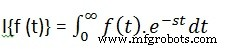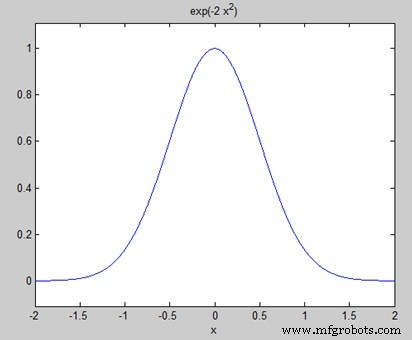﻿ MATLAB - 转换MfgRobots >> 亿迅智能制造网 >  >> Industrial programming >> MATLAB
MATLAB - 转换

MATLAB 提供了用于处理变换的命令，例如拉普拉斯变换和傅立叶变换。转换在科学和工程中用作简化分析和从另一个角度查看数据的工具。

MATLAB 提供了拉普拉斯 , 傅立叶fft 用于处理拉普拉斯、傅立叶和快速傅立叶变换的命令。

## 拉普拉斯变换`拉普拉斯(f(t))`

### 示例

`syms stab wlaplace(a)laplace(t^2)laplace(t^9)laplace(exp(-b*t))laplace(sin(w*t))laplace(cos( w*t))`

`ans =1/s^2ans =2/s^3ans =362880/s^10ans =1/(b + s) ans =w/(s^2 + w^2) ans =s/(s^2 + w^2)`

## 拉普拉斯逆变换

MATLAB 允许我们使用命令 ilaplace 计算逆拉普拉斯变换 .

`ilaplace(1/s^3)`

MATLAB 将执行上述语句并显示结果 -

`ans =t^2/2`

### 示例

`syms stab wilaplace(1/s^7)ilaplace(2/(w+s))ilaplace(s/(s^2+4))ilaplace(exp(-b*t) )ilaplace(w/(s^2 + w^2))ilaplace(s/(s^2 + w^2))`

`ans =t^6/720ans =2*exp(-t*w)ans =cos(2*t)ans =ilaplace(exp(-b*t), t, x) ans =sin(t*w)ans =cos(t*w)`

## 傅里叶变换

### 示例

`syms x f =exp(-2*x^2); %our functionezplot(f,[-2,2]) % 函数图FT =Fourier(f) % Fourier transform``FT =(2^(1/2)*pi^(1/2)*exp(-w^2/8))/2`

`ezplot(FT)`## 傅里叶逆变换

MATLAB 提供了傅立叶 用于计算函数的傅里叶逆变换的命令。例如，

`f =ifourier(-2*exp(-abs(w)))`

MATLAB 将执行上述语句并显示结果 -

`f =-2/(pi*(x^2 + 1))`

MATLAB